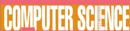# Analog Vs. Digital Computing - Research Article from World of Computer Science

This encyclopedia article consists of approximately 4 pages of information about Analog Vs. Digital Computing.
 This section contains 1,026 words (approx. 4 pages at 300 words per page) View a FREE sampleDigital computers calculate by manipulating binary digits (bits; ones and zeroes). Because bits are so simple to handle they can be made easily to stand for almost anything; hence the general usefulness of digital computers. Bits can symbolize words, instructions, laws of logic or of physics, numerical measurements, recorded images and sounds--anything that can be written down. In using a digital computer to solve a mathematical equation, for example, certain bits inside the computer are arranged to symbolize the constants and variables in the equation and others are arranged to symbolize the mathematical rules for manipulating those constants and variables. When the computer runs, it applies the rules to the variables just as a person would, only faster.

There is another quite different way to solve an equation: instead of symbolizing an equation, one can set up an experiment in...

(read more)

 This section contains 1,026 words (approx. 4 pages at 300 words per page) View a FREE sampleMore summaries and resources for teaching or studying Analog Vs. Digital Computing.
CopyrightsAnalog Vs. Digital Computing from Gale. ©2005-2006 Thomson Gale, a part of the Thomson Corporation. All rights reserved.